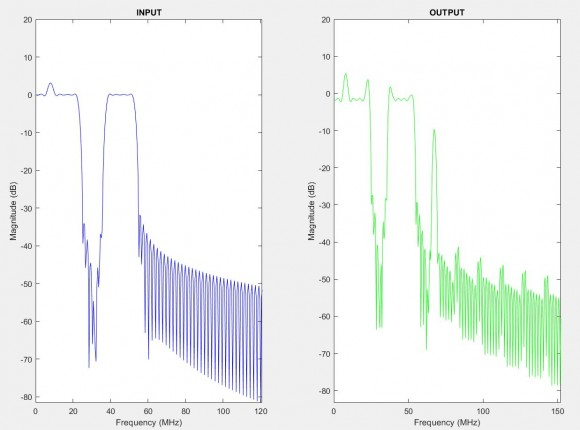## FFT Channelizer and PFBStarted by 4 years ago8 replieslatest reply 4 years ago520 views

Hello,

I am implementing a FFT/IFFT channelizer using a Polyphase Filter Bank, and I would like to have the advice regarding the results I get.

Here is the process I use:

SIGNAL DECIMATION -> PFB ANALYSIS -> FFT ==> IFFT -> PFB SYNTHESIS -> SIGNAL INTERPOLATION

Here are the input (before signal decimation) and the output (after signal interpolation) I have:I use a 256 decimation/interpolation and 256-points FFT/IFFT.

Do you have an idea of why I have an amplitude difference between the input and the output?

Thank you,

SW

[ - ]The analysis and synthesis filter response will govern the output.

[ - ]My problem is that I have, at least I think, the perfect reconstruction criterion, which is having the synthesis filters having the same coefficients as the analysis PFB but in the reverse order ... any advice?

thank you,

SW

[ - ]I would try the following:

1. Run only the analysis-synthesis filters (remove FFT-IFFT). If you get perfect replica, you know the filters are not the reason.

2. Remember, the FFT and IFFT can have different windowing, averaging, etc., so if 1. rules out the filters, I would focus on the FFT-IFFT details.

As a side, I am assuming you are only concerned about the pass-band being distorted in the output. The stop-band differences are surely because of the windowing of the FFT. Keep in mind, that rate-changing implies that you only care about the narrow-band/down-sampled portion of the output. Cascading the down-converter with the up-converter is not the typical use-case (though it can serve as a good test/validation case, I suppose)

[ - ]I have run the script taking away the FFT and the IFFT, I have the exact same output result, so the distortions are indeed due to the analysis/synthesis filter coefficients...

I wonder how come the polyphase decomposition does not end up in perfect reconstruction in my case

Thanks,

SW

[ - ]Please try to use the FFT for analysis and synthesis or for both the iFFT. I use this in my textbook and it works.

[ - ]Try my 60-path, non-maximally decimated, perfect reconstruction filter bank:

performs 30-to-1 down-sample, a binary mask (turn off subset of channels) and 1-to-30 up-sample.  Both input and output transforms are IFFT...

fred h

channelizer_60_x.m

you may also need myfrf... will attach it to next response

part copied below

% weights for 60-path analysis filter

h1=sinc((-299:299)/60.0).*kaiser(599,8.9)';

% weights for 60-path synthesis filter, 2-to-1 down sample

h2=remez(598,[0 0.75-0.04 1.25+0.04 30]/30,{'myfrf2',[1 1 0 0]},[1 1]);

hh1=reshape([0 h1],60,10);  % analysis coefficients

hh2=reshape([0 h2],60,10);  % synthesis coefficients

reg_hh1=zeros(60,20);    % 2-D analysis signal register

reg_hh2=zeros(60,20);    % 2-D synthesis signal register

u1=zeros(1,60)';

u2=zeros(1,60)';

u3=zeros(1,60)';

u4=zeros(1,60)';

u5=zeros(1,60)';

flg1=0;  % State machine input

flg2=0;  % state machine output

m1=1;

m2=0;

x0=[1 zeros(1,1999)];  % for impulse response... can change for any input

x8=zeros(1,2000);

gn=zeros(1,60)';            For binary mask between input and output channelizers

gn(1:16-1)=ones(1,16-1)';

gn(46+1:60)=ones(1,15-1)';

for nn=1:30:2000-20

u1(1:30)=fliplr(x0(nn:nn+29)).';

u1(31:60)=u1(1:30);

reg_hh1=[u1 reg_hh1(:,1:19)];

for kk=1:30

u2(kk)   =reg_hh1(kk,1:2:20)*hh1(kk,:)';

u2(kk+30)=reg_hh1(kk+30,2:2:20)*hh1(kk+30,:)';

end

if flg1==0

flg1=1;

elseif flg1==1

flg1=0;

u2=[u2(31:60);u2(1:30)];

end

u3=60*ifft(u2);

m1=m1+1;

u4=u3;

u4=u4.*gn;

%     u4(1:16)=u3(16:31);

%     u4(46:60)=u3(1:15);

u5=60*ifft(u4);

if flg2==0

flg2=1;

elseif flg2==1

flg2=0;

u5=[u5(31:60);u5(1:30)];

end

reg_hh2=[u5 reg_hh2(:,1:19)];

for kk=1:30

p1=reg_hh2(kk,1:2:20)*hh2(kk,:)';

p2=reg_hh2(kk+30,2:2:20)*hh2(kk+30,:)';

x8(m2+kk)=(p1+p2)/2;                  % output time series

end

m2=m2+30;

end

[ - ]here is myfrf called by channelizer_60_x

function [DH,DW] = myfrf(N, F, GF, W, A, diff_flag)

%---function [DH,DW] = remezfrf(N, F, GF, W, A, diff_flag)

%REMEZFRF Frequency Response Function for REMEZ.

%  REMEZ(N,F,A, ...) or

%  REMEZ(N,F,{'remezfrf',A}, ...) designs a linear-phase FIR filter

%  using REMEZ.

%

%  The symmetry option SYM defaults to 'even' if unspecified in the

%  call to REMEZ.

%

%   Copyright 1988-2002 The MathWorks, Inc.

% $Revision: 1.6$

%  modified by Karl Moerder and fred harris SDSU, copyright 2003

%  [DH,DW]=REMEZFRF(N,F,GF,W,A,diff_flag)

%      N: filter order (length minus one)

%      F: vector of band edges

%     GF: vector of interpolated grid frequencies

%      W: vector of weights, one per band

%      A: vector of amplitudes of desired frequency response at band edges F

% diff_flag: ==1 for differentiator (1/f) weights, ==0 otherwise

%

%     DH: vector of desired filter response (mag & phase)

%     DW: vector of weights (positive)

%

% NOTE: DH(GF) and DW(GF) are specified as functions of frequency

% Support query by REMEZ for the default symmetry option:

if nargin==2,

% Return symmetry default:

if strcmp(N,'defaults'),

DH = 'even';   % can be 'even' or 'odd'

return

end

end

if nargin < 6

diff_flag = 0;

else%+++

error('Differentiator option is not allowed with myfrf.')%+++

end

% Prevent discontinuities in desired function

for k=2:2:length(F)-2

if F(k) == F(k+1)

end

end

if length(F) ~= length(A)

error('Frequency and amplitude vectors must be the same length.')

end

nbands = length(A)/2;

l = 1;

while (l+1)/2 <= nbands

sel = find( GF>=F(l) & GF<=F(l+1) );

% desired magnitude is line connecting A(l) to A(l+1)

if F(l+1)~=F(l)   %

slope=(A(l+1)-A(l))/(F(l+1)-F(l));

DH(sel) = polyval([slope A(l)-slope*F(l)],GF(sel));

else   % zero bandwidth band

DH(sel) = (A(l)+A(l+1))/2;

end

%---DW(sel) = W((l+1)/2) ./ (1 +(diff_flag & A(l+1) >= .0001)*(GF(sel)/2 - 1));

if A(l+1) > 0.0001;%+++ check for passband or stopband

DW(sel) = W((l+1)/2);%+++ passband

else;%+++

DW(sel) = W((l+1)/2) * (GF(sel)/GF(sel(1)));%+++ stopband

end;%+++

l = l + 2;

end

[ - ]